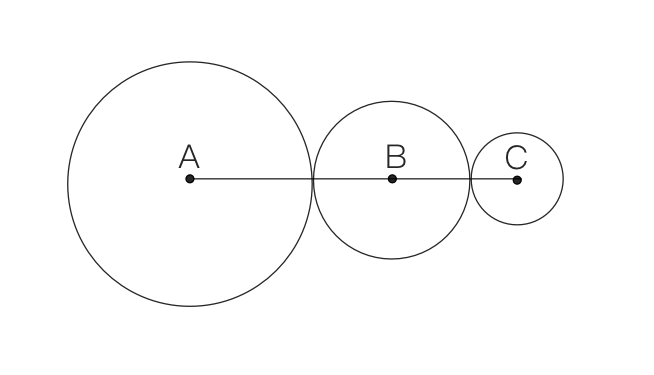### Still have math questions?

In the figure, the radius of circle A is twice the radius of circle B and four times the radius of circle C. If the sum of the circumferences of the three circles is $$42\pi$$, find the measure of AC.